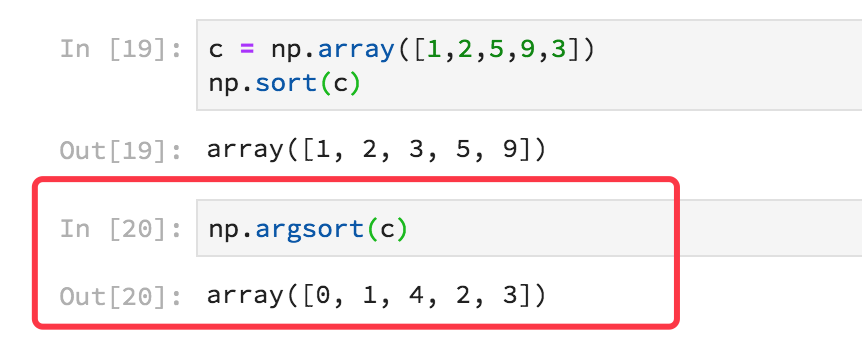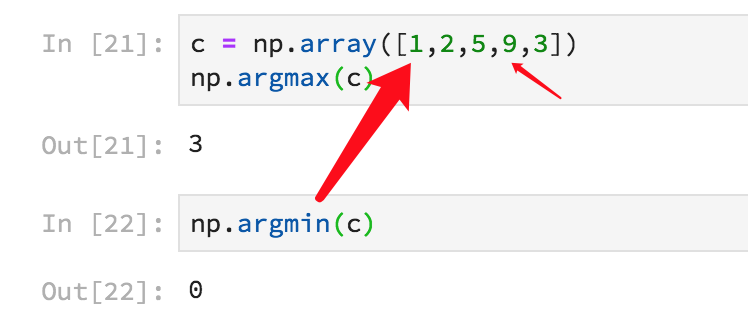• 当然要先引入numpy包import numpy as npList转numpy.array:temp = np.array(list) numpy.array转List:arr = temp.tolist() 原来是打算使用这种转换直接编辑OpenCV中的Mat类，后来发现不用转换，可以直接将一个Mat类...
当然要先引入numpy包
import numpy as np
List转numpy.array:
temp = np.array(list) 
numpy.array转List:
arr = temp.tolist() 
原来是打算使用这种转换直接编辑OpenCV中的Mat类，后来发现不用转换，可以直接将一个Mat类当做三维数组来提到其中的每一个像素。同时按照这种方式转换的类型，opencv输出会是一张黑图，所以放弃。
展开全文python
• 这3个函数在我用numpy实现knn时候用得到。虽然我是用的别的函数慢慢做的，也是可以解决的，但是这3个实在是很合适。所以有必要学习一下。 1.argsort函数 sort我们都知道，就是排序： c = np.array([1,2,5,9,3]) np....
在讲这个方法前，我想先讲几个函数，如果只需要标题的方法，请前往第四部分。
这3个函数在我用numpy实现knn时候用得到。虽然我是用的别的函数慢慢做的，也是可以解决的，但是这3个实在是很合适。所以有必要学习一下。
1.argsort函数
sort我们都知道，就是排序：
c = np.array([1,2,5,9,3])
np.sort(c)

array([1, 2, 3, 5, 9])

arg就是下标的排序：可以看到返回的是对应排序的下标或者是叫索引。
2.argmax与argmin.
这两个就很好理解了。一个返回最大值的下标，一个返回最小值的索引。3.bincount
说真的，这个我以前从没用过。和列表list.count(a)统计a在列表中出现的次数很像，但又不同。 不过还是很简单的。看一个例子就懂：它返回的是0–序列最大值在这个array中出现的次数。 例如第一个0，在array中没有出现，就是0。1-3都出现了1次，所以是1。9出现了3次，所以返回的最后一个位置就是9出现的次数-----9。
4 求array中出现次数最多的元素
有了上面的知识，这个问题就很简单了。一句话：
c = np.array([1,2,5,9,9,9,3])
d = np.argmax(np.bincount(c))
d

9

list(c).index(d)#下标
3

展开全文• ## numpy中array和asarray的区别

万次阅读 多人点赞 2017-02-22 14:45:06
array和asarray都可以将结构数据转化为ndarray，但是主要区别就是当数据源是ndarray时，array仍然会copy出一个副本，占用新的内存，但asarray不会。 举例说明： import numpy as np #example 1: data1=[[1,1,1],...
array和asarray都可以将结构数据转化为ndarray，但是主要区别就是当数据源是ndarray时，array仍然会copy出一个副本，占用新的内存，但asarray不会。
举例说明：

import numpy as np

#example 1:
data1=[[1,1,1],[1,1,1],[1,1,1]]
arr2=np.array(data1)
arr3=np.asarray(data1)
data1=2
print 'data1:\n',data1
print 'arr2:\n',arr2
print 'arr3:\n',arr3

输出：

data1:
[[1, 1, 1], [1, 2, 1], [1, 1, 1]]
arr2:
[[1 1 1]
[1 1 1]
[1 1 1]]
arr3:
[[1 1 1]
[1 1 1]
[1 1 1]]
可见array和asarray没有区别，都对元数据进行了复制。

import numpy as np

#example 2:
arr1=np.ones((3,3))
arr2=np.array(arr1)
arr3=np.asarray(arr1)
arr1=2
print 'arr1:\n',arr1
print 'arr2:\n',arr2
print 'arr3:\n',arr3


输出：

arr1:
[[ 1.  1.  1.]
[ 2.  2.  2.]
[ 1.  1.  1.]]
arr2:
[[ 1.  1.  1.]
[ 1.  1.  1.]
[ 1.  1.  1.]]
arr3:
[[ 1.  1.  1.]
[ 2.  2.  2.]
[ 1.  1.  1.]]
此时两者才表现出区别


展开全文python
• 一、PIL.Image 转 numpy.array from PIL import Image import numpy as np Img = Image.open('G:/Experiment/train/images/000_HC.png') array_img = np.array(Img) 二、numpy.array 转 PIL.Image img = ...
一、PIL.Image 转 numpy.array
from PIL import Image
import numpy as np

Img =  Image.open('G:/Experiment/train/images/000_HC.png')
array_img = np.array(Img)

二、numpy.array 转 PIL.Image
img = Image.fromarray(translation_img4.astype('uint8')).convert('RGB') #  numpy.array 转 PIL.Image

# im = Image.fromarray(img) 直接这样发现没有啥区别，不懂

Image._show(img)

展开全文• 共同作用：将原数组或numpy格式数组拷贝一份，或选其中部分元素当原数据是numpy格式时，asarray为取其中的部分数据（不拷贝），array为拷贝原数据部分内容如：import numpy as np #example 1: data1=[[1,1,1],...
• 1、ndarray转换成matriximport numpy as np from numpy import random,matr_arr=random.rand(4,4) print('r_arr',r_arr) ...print(r_mat.I)#逆运行结果：r_arr [[ 0.65603592 0.39908438 0.44722351 0.926527
• Numpy中的矩阵乘法分为两大情况，使用numpy.array和使用numpy.matrix. Numpy确实重载了*操作符，可以直接对array或者matrix对象进行乘法运算，但是在不同对象上，其意义是有区别的。 对于array对象 *（或multiply）...
• 下面小编就为大家分享一篇对numpyarray和python中自带的list之间相互转化详解，具有很好的参考价值，希望对大家有所帮助。一起跟随小编过来看看吧
• a=[1,2,3,4]将list转化为numpy.array: a.tolist()将numpy.array 转为list numpy.array(a)
• 文章目录Numpy与Python内置数据类型list的异同Numpy.arrayNumpy.matrix的异同Numpy.ndarray的主要特性Numpy.ndarray的主要操作 Numpy与Python内置数据类型list的异同 Python 本身是设计为通用编程语言的，在科学...
• 主要介绍了python求numpyarray按列非零元素的平均值案例，具有很好的参考价值，希望对大家有所帮助。一起跟随小编过来看看吧
• #coding:utf-8 import timeitcommon_for = """ for d in data: s += d ""...common_numpy_sum = """ numpy.sum(data) """def timeit_lipython
• 如下所示： import numpy a = numpy.array(([3,2,1],[2,5,7],[4,7,8])) ... 您可能感兴趣的文章:python中找出numpy array数组的最值及其索引方法对numpy中数组元素的统一赋值实例Python numpy 常用函数总结
• ## numpy对array索引

万次阅读 2018-06-14 15:03:42
numpyarray索引对numpyarray索引时总是容易出错，借此机会总结一下numpyarray最常用的索引方法1.单个元素的索引In :#1.1.一维array,单个元素的索引使用整数 import numpy as np a = np.array([3, 5, 1, 2,...
• ## Python-Numpy中Array用法总结

万次阅读 多人点赞 2018-10-14 19:45:12
Numpyarray（数组） Numpy主要对象是齐次多维数组，由正整数元组索引，Numpy中维度称为轴（axis），数组的维数称为秩（rank）。 可以参考：Numpy快速入门 1.1 创建数组 常规方法创建数组 import numpy as np #一...Python
• #-*- encoding:utf-8 -*- import numpy import io from io import StringIO #import io.StringIO ...def createArray(): d = numpy.zeros((3,4)) print(d.dtype) print(d.dtype.itemsize) oneMatrix =python
• Numpy是专门针对数组的操作运算进行了设计，所以数组的存储效率输入输出性能远优于Python中的嵌套列表，数组越大，Numpy的优势就越明显。通常Numpy数组中的所有元素的类型都是相同的，而Python列表中的元素类型......